#### Polarization of Light, Physics tutorial

Introduction to polarization of light:

Polarization is the property of light or other electromagnetic radiation, which is primarily understood via studying the waves of the radiation. It was introduced by Etienne Louis Malus, a French physicist in the year of 1800. The visible light is the range of electromagnetic radiation which humans can see, and its wavelengths encompass a range from around 380 to 740 nanometers. The electromagnetic radiation is a radiation which is generated through electric and magnetic fields which travel altogether at the speed of light via space.

Polarization is as well generated naturally in certain instances, like if light passes via specific crystals or via artificial material designed to make this effect. Polarized sunglasses, for illustration, work via only letting vertical polarized light in. They are popular among outdoors enthusiasts and people who want to decrease the glare from the sun.

Radio transmission and receiver antennas as well are polarized, and one of the most general uses of this property is in the radar. AM and FM radios make use of vertical polarization as televisions make use of horizontal polarization. Interestingly, such two directions alternate by the use of satellite communications - even for television use. A satellite can carry two different transmissions of a frequency and double the amount of customers which can be served.

Definition: Light can be explained as an electromagnetic wave that, similar to radio waves, propagates through a sinusoidal oscillation of the electric field. The direction in which the electric field oscillates as it propagates is termed as the polarization.

Types of polarization:

Based on how the electric field is oriented, we categorize polarized light into three kinds of polarizations:

1) Plane or linear Polarization: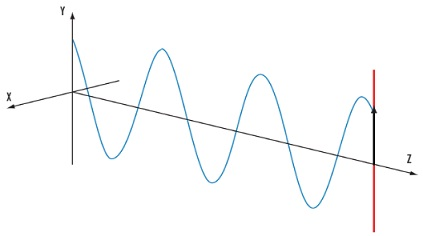The electric field of light is confined to the single plane all along the direction of propagation. A beam linearly polarized all along the x-axis and traveling in the positive z-direction can be symbolized by:

E = Ex + Ey = (x + y) A sin (kz - ωt)

2) Circular polarization: The electric field of light comprises of two linear components which are perpendicular to one other, equivalent in amplitude, however encompass a phase difference of π/2. The resultant electric field rotates in a circle around the direction of propagation and, based on the rotation direction, is termed left or right-hand circularly polarized light.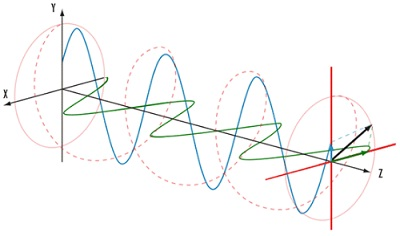Assume that the two components have equivalent amplitudes again; however now consider the case where these two components are not in phase, in such a way that the angles of the sine functions are different. In specific, assume that there is a constant phase difference of π/2 between them that corresponds to a distance of π/4 in the fixed time picture. The x-component is:

Ex = x A sin (kz - ωt - π/2)

As the y-component is as before:

Ey = y A sin (kz - ωt)

3) Elliptical Polarization: In this, the electric field of light illustrates an ellipse. These outcomes from the combination of two linear components having differing amplitudes and/or a phase difference that is not π/2. This is the most common explanation of polarized light and circular and linear polarized light can be observed as special cases of elliptically polarized light.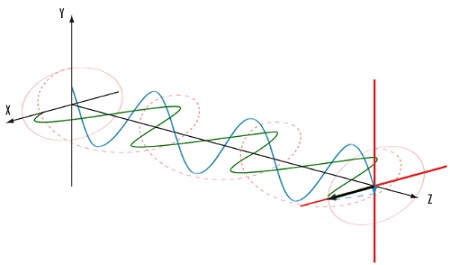All of the above states of polarization illustrated above are in reality special cases of the most general state of polarization, termed as elliptical polarization, in which the tip of the electric field vector E traces out an ellipse in the x-y plane. The two components might encompass unequal amplitudes Ax ≠ Ay, and as well might have a different relative phase, often represented by δ. That is, we might write usually:

Ex = x Ax sin (kz - ωt + δ)

Whereas, the y component is as:

Ey = y Ay sin (kz - ωt)

Malus's Law:

Assume that the polarized light of intensity I(0) incident on an ideal linear polarizer. Suppose the transmission axis of this polarizer be at an angle of θ with respect to the initial polarization. Merely light having its electric field vector parallel to the axis of the polarizer will be transmitted via the polarizer.

The intensity of the light coming out from the second polarizer is represented by the Malus' Law:

I(θ) = I(0) cos2(θ).

Now, consider the condition where light from the first polarizer is incident on the second polarizer whose transmission axis is at an angle of ψ with respect to the initial polarization. The intensity of the light emerging from the second polarizer is represented by:

I(ψ) = I(0) cos2(θ) cos2(θ - ψ).

Degree of Polarization:

The degree of polarization is fundamentally a measure of the extent to which the light we are studying is polarized and is represented by:

V = (Imax - Imin)/(Imax + Imin)

Here, Imin and Imax are the minimum and maximum intensities of the light, correspondingly. For partially polarized light, this can as well be represented as:

V = Ip/(Ip + Iup)

Here, Ip is the intensity of the polarized part and Iup is the intensity of the unpolarized part. At times V is termed as the fringe visibility.

Polarization by Reflection: Brewster's Law

Whenever linearly polarized light is incident on the plane surface, the amount of light reflected, the reflectance (R), based on the angle of incidence and the orientation of the polarization vector relative to the scattering plane. This is explained through the Fresnel equations:

R|| = |[tan(θi - θt)/tan(θi + θt)]|2

R = |[sin(θi - θt)/sin(θi + θt)]|2

One effect of the equation above is that when θi + θt = π/2, tan (θi + θt) → ∞ and Rk → 0. This takes place if θi = θB where θB is termed as Brewster's angle and θt = β. Replacing θB and β into the second equation, subject to θB +β = π/2 leads to:

tan θB = nt/no

At θB merely light having its E-field perpendicular to the scattering plane will be reflected. As a effect, it is possible to generate polarized light from Unpolarized light by letting it strike an interface at Brewster's angle.

Changing the polarization of light:

The Unpolarized light can be polarized by employing a polarizer or polarizing beamsplitter, and the state of already polarized light can be modified by employing a polarizer and/or optical components that are birefringent. In this part we look at certain illustrations of these kinds of components.

a) Polarizer and polarizing beamsplitter:

A polarizer transmits just a single orientation of the linear polarization, and blocks the rest of the light. For illustration: a polarizer oriented all along x passes Ex and blocks Ey.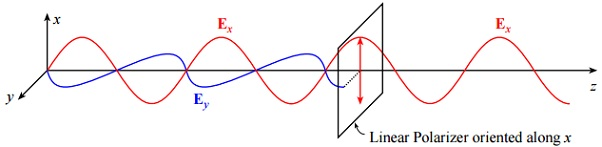A few polarizers remove the non-passed polarization component (that is, Ey in the above illustration) by absorbing it, whereas others reflect this component. Absorbing polarizer's is convenient if it is desirable to fully remove one polarization component from the system. A demerit of absorbing polarizer is that they are not extremely durable and might be damaged through high intensity light (that is, as found in numerous laser applications).

b) Birefringence:

Several materials encompass a different index of refraction for light polarized all along different directions. This phenomenon is termed as birefringence. For illustration, assume that a light polarized all along the x direction notices an index of nx, as light polarized all along the y direction sees an index ny. Now assume that linearly polarized light passes via a piece of such a material of length L, here the linear polarization axis is oriented at 45° with respect to the x and y axes. The fixed time picture therefore looks similar to: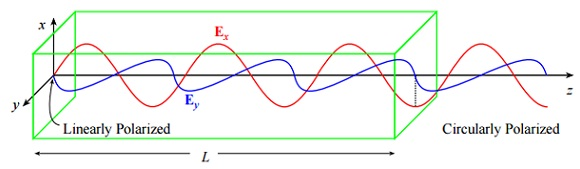Applications of polarization of light:

1) Implementing polarization control can be helpful in imaging the applications. By putting a linear polarizer over the light source, the lens or both, it is possible to remove glare and hot spots from the reflective objects or bring out surface defects.

2) Material stress can be computed in transparent objects by employing the photoelastic effect. Stressed material becomes birefringent, and the stress and its associated birefringence can be evaluated by employing the polarized light.

3) Polarization is as well extremely significant in the chemical, pharmaceutical and food and beverage industries. Most of the significant chemical compounds, like active pharmaceutical ingredients or sugar, are 'optically active' and rotate the polarized light. The amount of rotation is found out by the nature and the concentration of the compound, letting polarimetry to detect and quantify such compounds.

Tutorsglobe: A way to secure high grade in your curriculum (Online Tutoring)

Expand your confidence, grow study skills and improve your grades.

Since 2009, Tutorsglobe has proactively helped millions of students to get better grades in school, college or university and score well in competitive tests with live, one-on-one online tutoring.

Using an advanced developed tutoring system providing little or no wait time, the students are connected on-demand with a tutor at www.tutorsglobe.com. Students work one-on-one, in real-time with a tutor, communicating and studying using a virtual whiteboard technology.  Scientific and mathematical notation, symbols, geometric figures, graphing and freehand drawing can be rendered quickly and easily in the advanced whiteboard.

Free to know our price and packages for online physics tutoring. Chat with us or submit request at info@tutorsglobe.com